ET大脑

API与工具

# 日志分析

## 概述

DRDS SQL 审计与分析，依托日志服务产品，提供强大的日志分析能力。本文档提供常见场景的 SQL 日志分析语句及示例。

## 注意事项

• 序号1部分的语句为默认过滤条件。
• 序号2部分的语句为追加的过滤条件。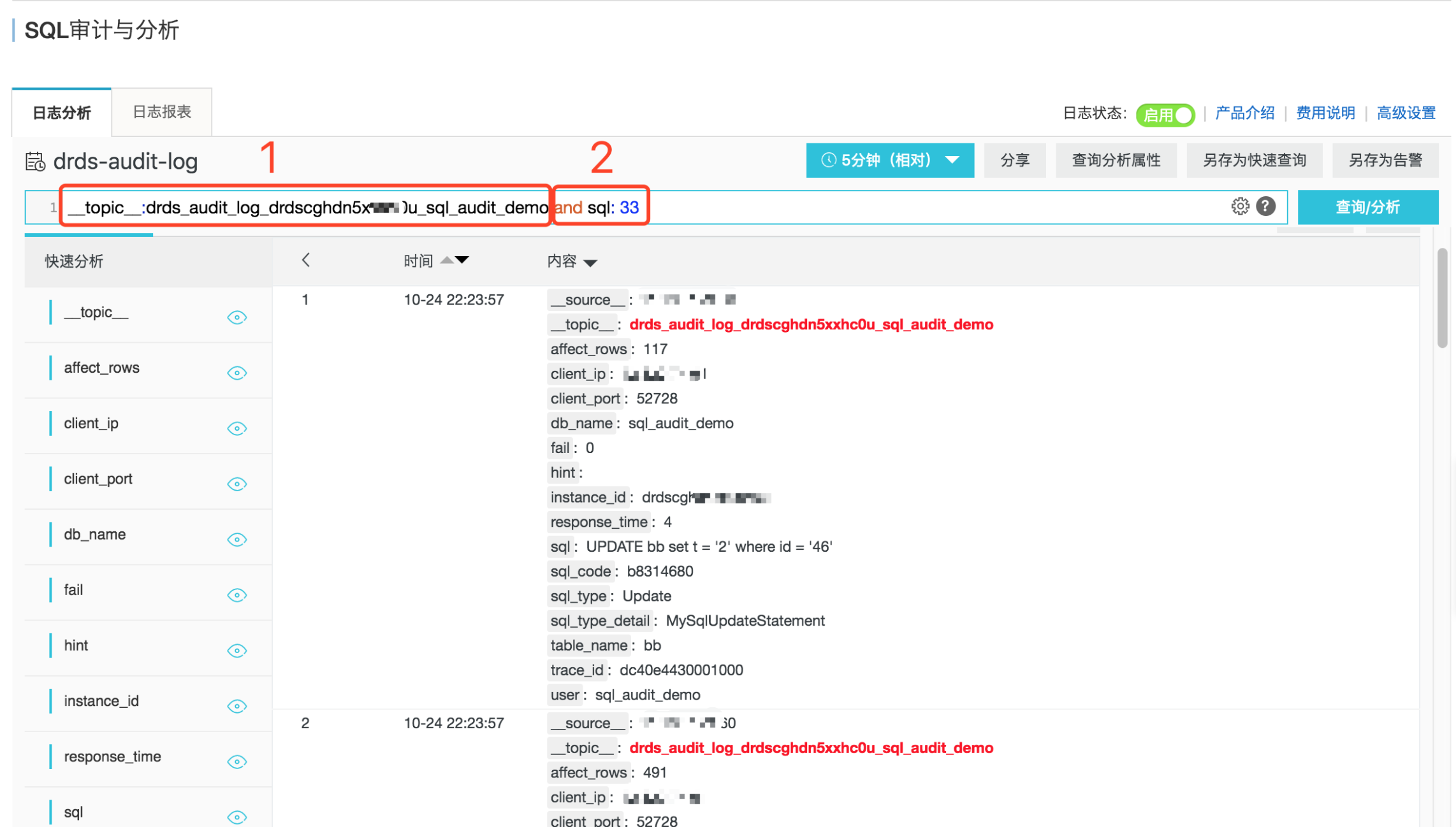## 快速定位问题 SQL

#### 模糊搜索

`` and sql: 34``#### 字段搜索

``and sql_type:Drop``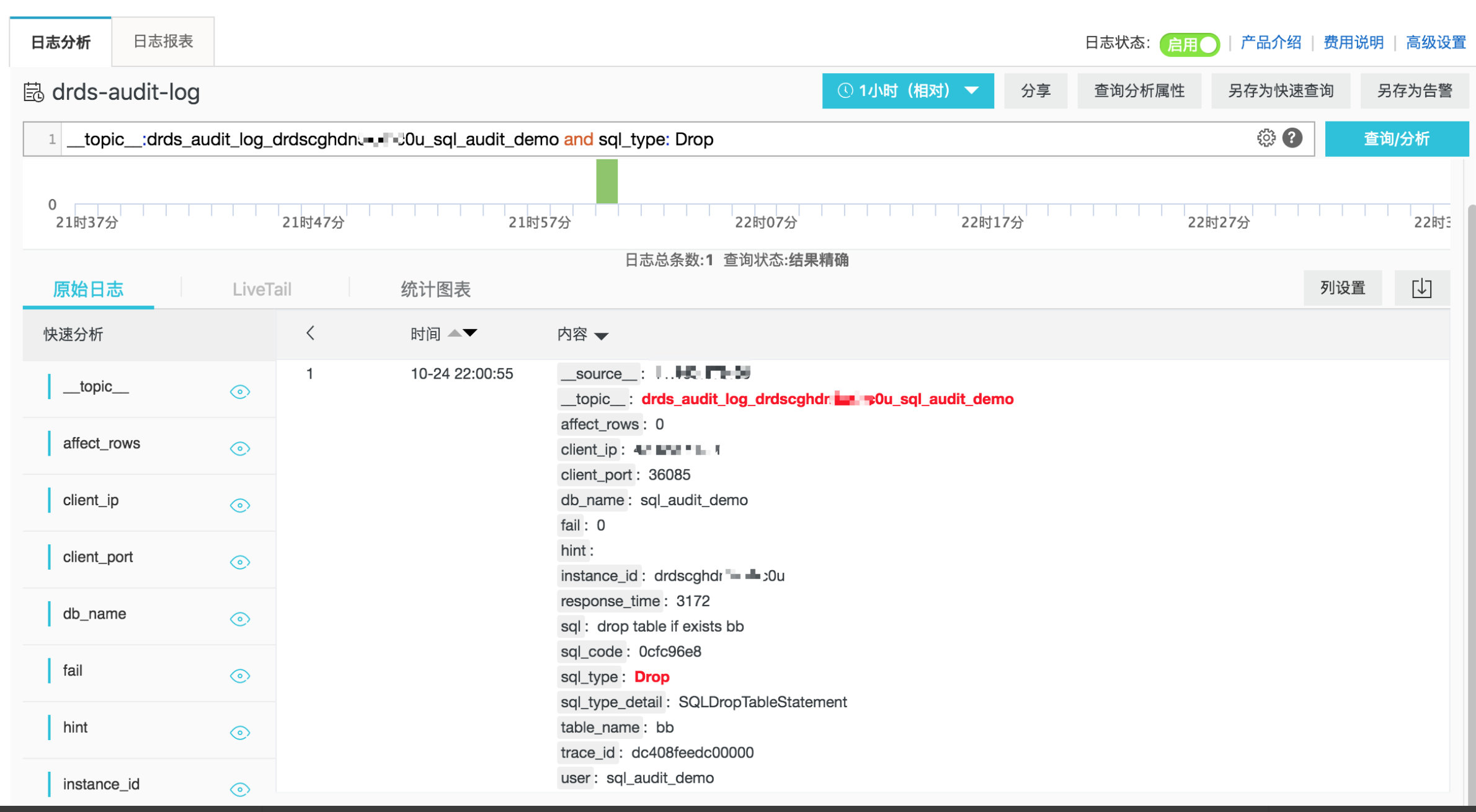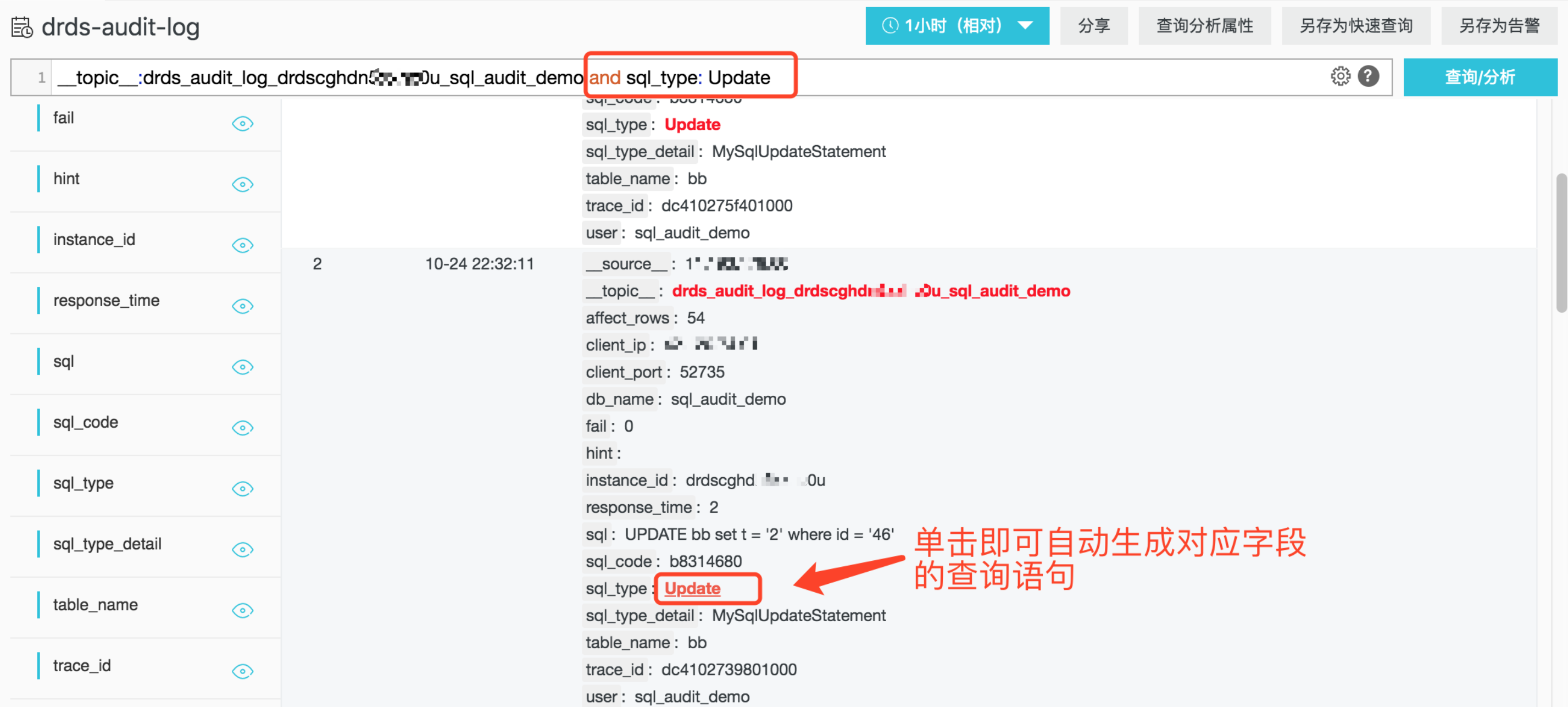#### 多条件搜索

``and sql:34 and sql_type: Delete``

#### 数值比较搜索

`` and response_time  > 1507 and sql_type: Insert``

`` and affect_rows  > 100 and sql_type: Delete``

## SQL 执行状况分析

#### SQL 执行失败率

``| SELECT sum(case when fail = 1 then 1 else 0 end) * 1.0 / count(1) as fail_ratio``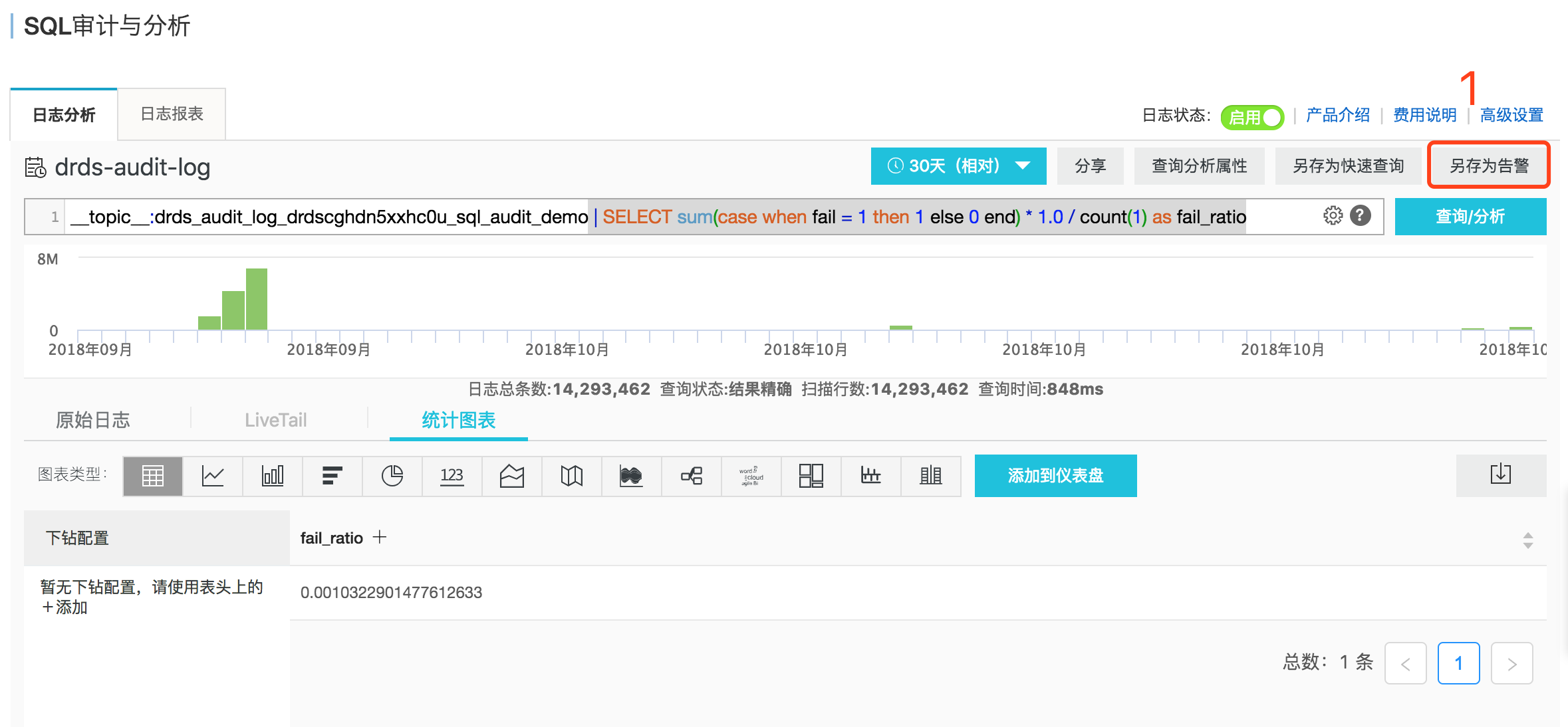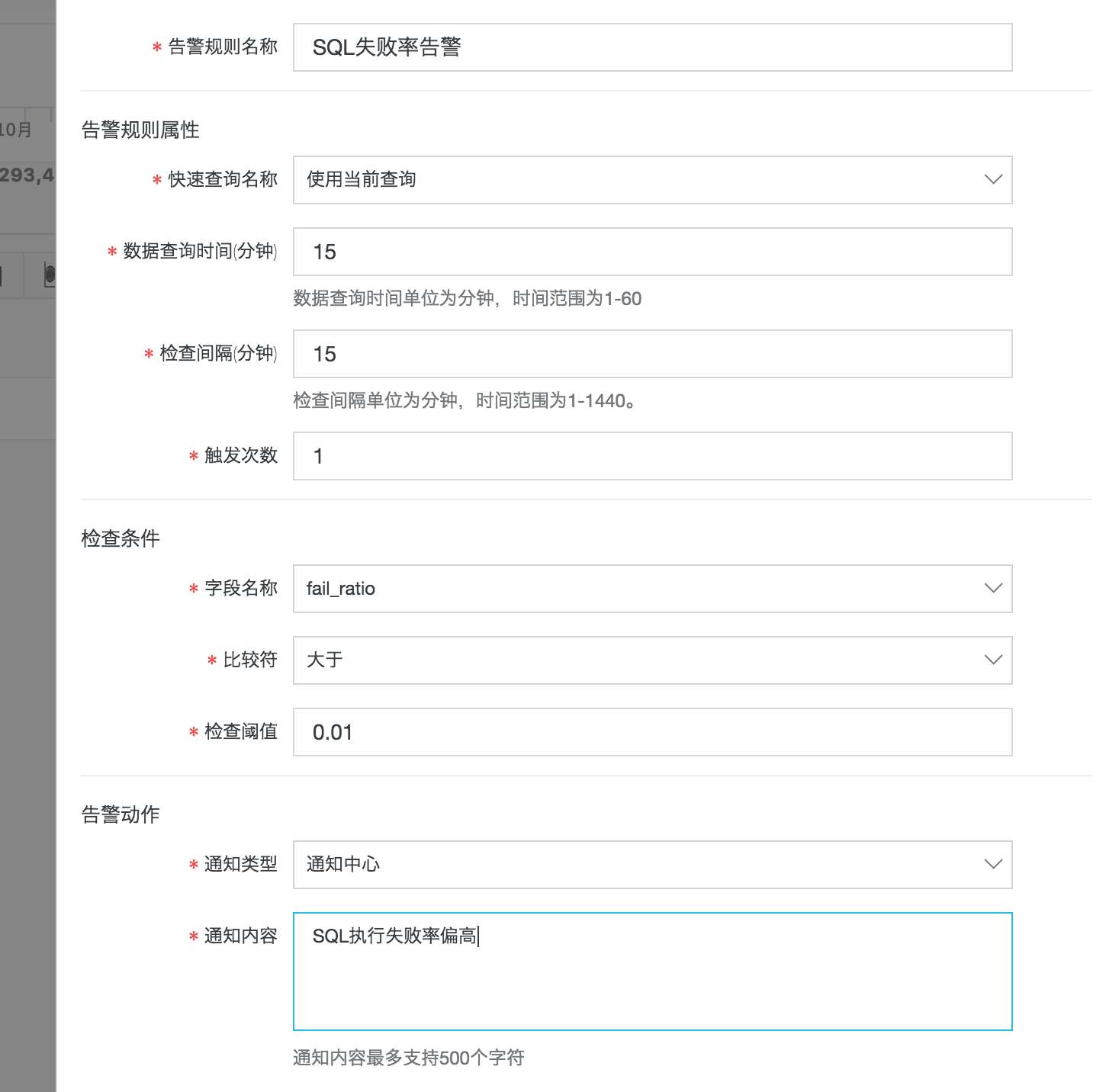#### SQL 累计查询行数

``  and sql_type: Select | SELECT sum(affect_rows)``

#### SQL 类型分布

``  | SELECT  sql_type, count(sql) as times GROUP BY sql_type``

#### SQL 独立用户 IP 分布

`` | SELECT  user, client_ip, count(sql) as times GROUP BY user, client_ip``

## SQL 性能分析

#### SELECT 平均耗时

`` and sql_type: Select | SELECT avg(response_time)``

#### SQL 执行耗时分布

`` and response_time > 0 | select   case  when response_time <= 10 then '<=10毫秒'  when response_time > 10 and response_time <= 100 then '10~100毫秒'  when response_time > 100 and response_time <= 1000 then '100毫秒~1秒'  when response_time > 1000 and response_time <= 10000  then '1秒~10秒'  when response_time > 10000 and response_time <= 60000  then '10秒~1分钟'  else '>1分钟' end as latency_type,  count(1) as cnt group by latency_type order by latency_type DESC``

#### 慢 SQL Top 50

`` | SELECT date_format(from_unixtime(__time__), '%m/%d %H:%i:%s') as time, user, client_ip, client_port, sql_type, affect_rows, response_time, sql ORDER BY  response_time desc LIMIT 50``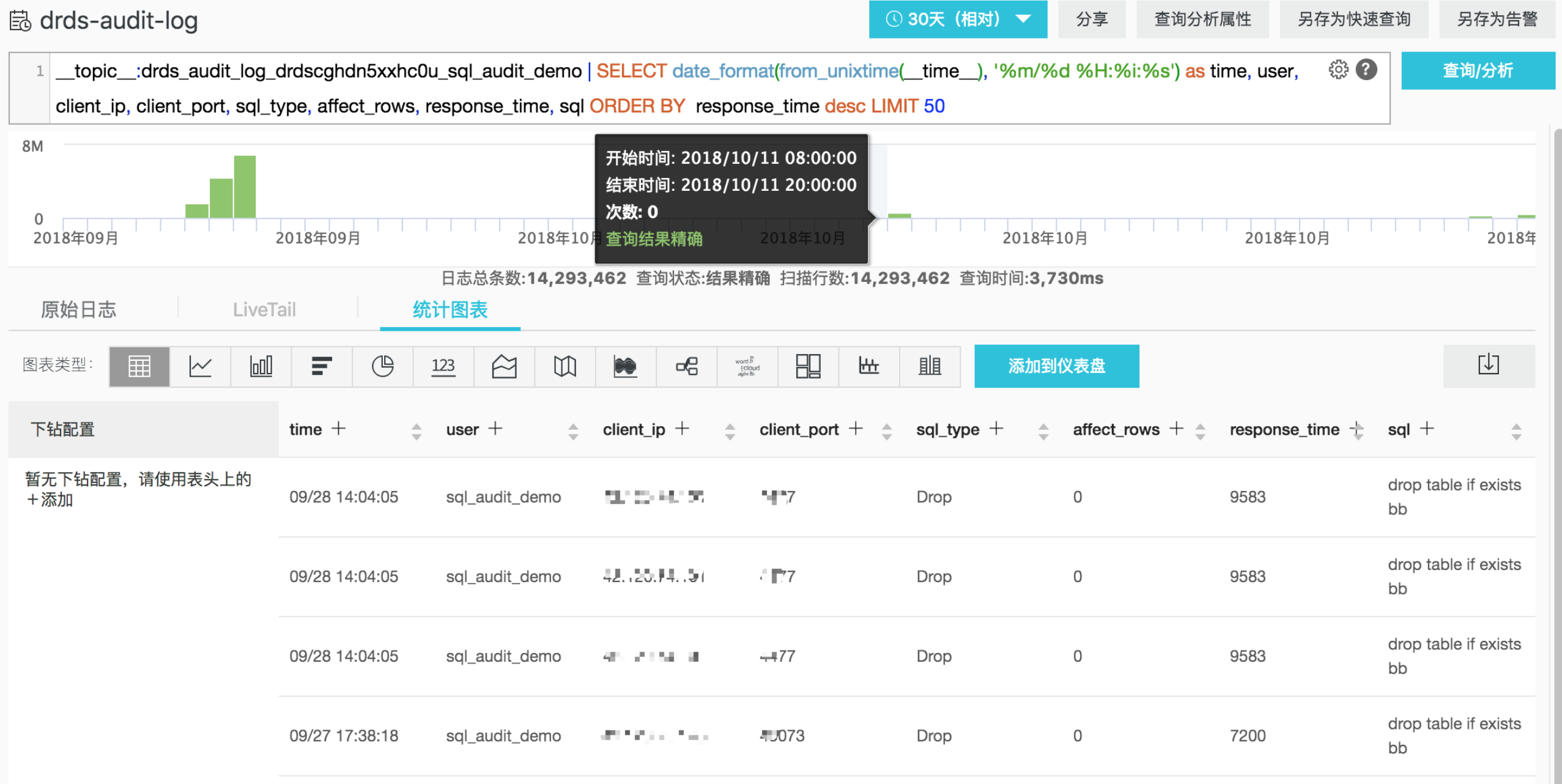#### 高代价 SQL 模板 Top 10

``| SELECT sql_code as "SQL模板ID", round(total_time * 1.0 /sum(total_time) over() * 100, 2) as "总体耗时比例(%)" ,execute_times as "执行次数", round(avg_time) as "平均执行时间",round(avg_rows) as "平均影响行数", CASE WHEN length(sql) > 200 THEN  concat(substr(sql, 1, 200), '......') ELSE trim(lpad(sql, 200, ' ')) end as "样例SQL" FROM  (SELECT sql_code, count(1) as execute_times, sum(response_time) as total_time, avg(response_time) as avg_time, avg(affect_rows) as avg_rows, arbitrary(sql) as sql FROM log GROUP BY sql_code) ORDER BY "总体耗时比例(%)" desc limit 10``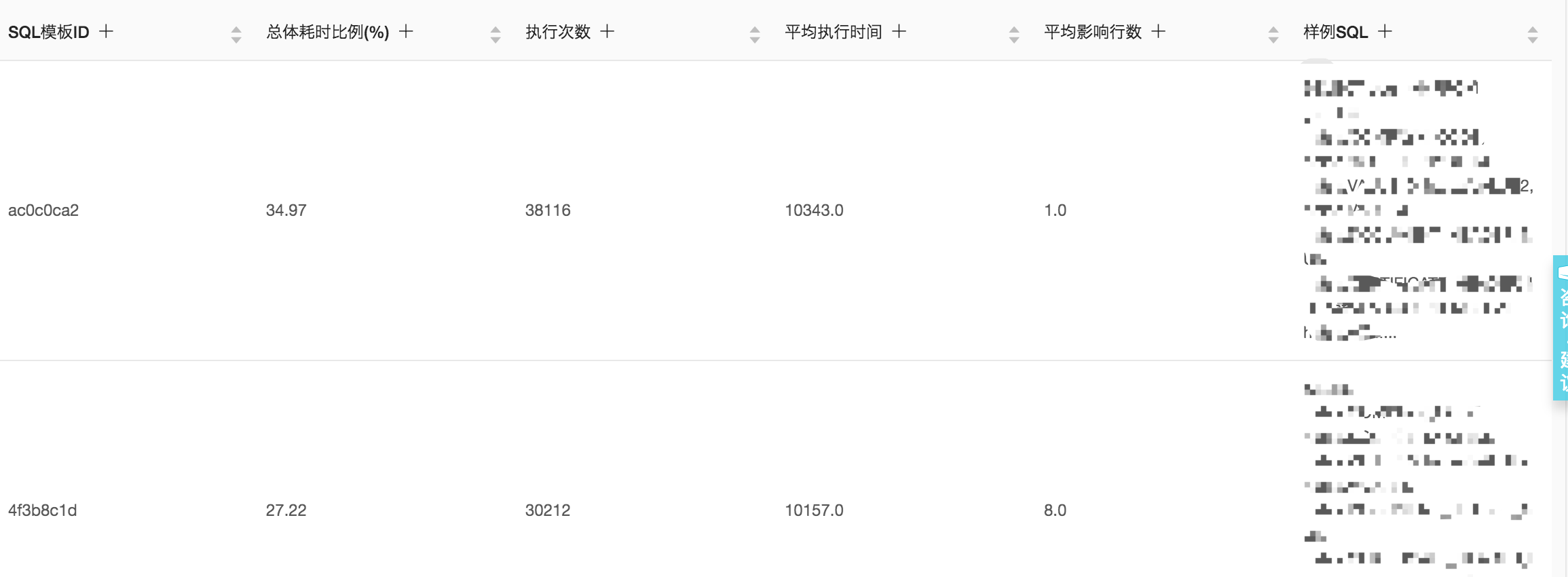#### 事务平均执行时长

`` | SELECT  sum(response_time) / COUNT(DISTINCT substr(trace_id, 1, strpos(trace_id, '-') - 1)) where strpos(trace_id, '-') > 0``

#### 慢事务 Top 10

``| SELECT substr(trace_id, 1, strpos(trace_id, '-') - 1) as "事务ID" , sum(response_time) as "事务耗时" where strpos(trace_id, '-') > 0 GROUP BY substr(trace_id, 1, strpos(trace_id, '-') - 1) ORDER BY "事务耗时" DESC LIMIT 10``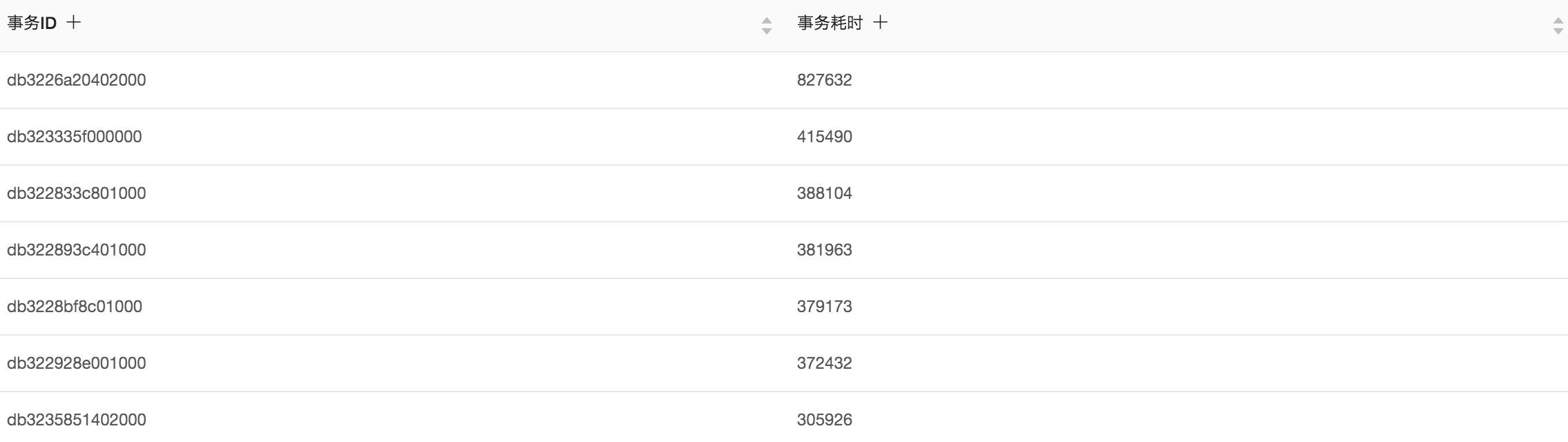`` and trace_id: db3226a20402000*``
##### 大批量操作事务 Top 10

``| SELECT substr(trace_id, 1, strpos(trace_id, '-') - 1) as  "事务ID" , sum(affect_rows) as "影响行数" where strpos(trace_id, '-') > 0 GROUP BY substr(trace_id, 1, strpos(trace_id, '-') - 1) ORDER BY "影响行数" DESC LIMIT 10``

## SQL 安全性分析

#### 错误 SQL 类型分布

`` and fail > 0 | select sql_type, count(1) as "错误次数" group by sql_type``

#### 高危 SQL 列表

`` and sql_type: Drop OR  sql_type: Truncate``

#### 大批量删除 SQL 列表

`` and affect_rows > 100 and sql_type: Delete | SELECT date_format(from_unixtime(__time__), '%m/%d %H:%i:%s') as time, user, client_ip, client_port, affect_rows, sql ORDER BY  affect_rows desc LIMIT 50``